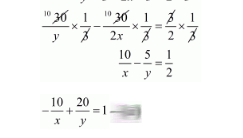# A takes 3 hours more than B to walk a distance of 30 km.Question:

A takes 3 hours more than $B$ to walk a distance of $30 \mathrm{~km}$. But, if $A$ doubles his pace (speed) he is ahead of $B$ by $1 \frac{1}{2}$ hours. Find the speeds of $A$ and $B$.

Solution:

Let the speed of A and B be x Km/hr and y Km/hr respectively. Then,

Time taken by A to cover $30 \mathrm{~km}=\frac{30}{x} h r s$,

And, Time taken by B to cover $30 \mathrm{~km}=\frac{30}{y} h r s$.

By the given conditions, we have

$\frac{30}{x}-\frac{30}{y}=3$

$\frac{10}{x}-\frac{10}{y}=1 \cdots(i)$

If A doubles his pace, then speed of A is $2 x \mathrm{~km} / \mathrm{hr}$

Time taken by A to cover $30 \mathrm{~km}=\frac{30}{2 x} \mathrm{hrs}$,

Time taken by B to cover $30 \mathrm{~km}=\frac{30}{y} h r s$.

According to the given condition, we have

$\frac{30}{y}-\frac{30}{2 x}=1 \frac{1}{2}$

$\frac{30}{y}-\frac{30}{2 x}=\frac{3}{2}$

$\frac{30}{y} \times \frac{1}{3}-\frac{30}{2 x} \times \frac{1}{3}=\frac{3}{2} \times \frac{1}{3}$Putting $\frac{1}{y}=\|$ and $\frac{1}{y}=v$, in equation (i) and (ii), we get

$10 u-10 v=1$

$10 u-10 v-1=0 \cdots(i i i)$

$-10 u+20 v=1$

$-10 u+20 v-1=0 \cdots(i v)$

Adding equations (iii) and (iv), we get,

$10 v-2=0$

$10 v=2$

$v=\frac{2}{10}$

$v=\frac{1}{5}$

Putting $v=\frac{1}{5}$ in equation (iii), we get

$10 u-10 v-1=0$

$10 u-10 \times \frac{1}{5}-1=0$

$10 u-2-1=0$

$10 u-3=0$

$10 u=3$

$u=\frac{3}{10}$

Now, $u=\frac{3}{10}$

$\frac{1}{x}=\frac{3}{10}$

$x=\frac{10}{3}$

and, $v=\frac{1}{5}$

$\frac{1}{y}=\frac{1}{5}$

$y=5$

Hence, the A's speed is $\frac{10}{3} \mathrm{~km} / \mathrm{hr}$,

The B's speed is $5 \mathrm{~km} / \mathrm{hr}$.